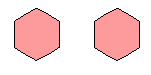Email us to get an instant 20% discount on highly effective K-12 Math & English kwizNET Programs!

#### Online Quiz (WorksheetABCD)

Questions Per Quiz = 2 4 6 8 10

### MEAP Preparation - Grade 7 Mathematics1.145 Geometry Part Three - Review Test

 Q 1: Solids have curved surfaces and some solids have flat surfaces. A flat surface on a solid is called aedgefacevertex Q 2: The formula for area of a rectangle islength + widthlength x widthlength - width Q 3: Is this figure symmetric?NoYes Q 4: ____ are ordered pairs of numbers written within parentheses and used to locate points on coordinate axes.coordinate gridcoordinatesordered number pairs Q 5: In the figure name the location or ordered pair of point C.(5,3)(5,4)(1,3) Q 6: What is the area of a rectangle whose length is 27 feet and width is 24 feetArea = 27 x 24 = 648 sq feetArea = 27 + 24 = 51 sq feetArea = 27 - 24 = 3 sq feet Q 7: Two figures are similar when they havesame shape and same sizesame shape but not necessarily same size Q 8: What is the perimeter of a rectangle with length 8 cm and width 7 cm?50 cm56 cm30 cm15 cm Question 9: This question is available to subscribers only! Question 10: This question is available to subscribers only!